Demo problem: Relaxation oscillations of an interface between two viscous fluids in an axisymmetric domain

In an earlier example we considered a free surface Navier–Stokes problem in which the interface between two viscous fluids was deformed to a prescribed shape and then allowed to relax. This example considers a similar problem in which the two fluids occupy a cylindrical domain which is orientated such that its axis of symmetry is normal to the equilibrium position of the interface. The problem is assumed to be axisymmetric and so the computational domain is rectangular as before, with the difference here that the governing equations are the axisymmetric Navier–Stokes equations as opposed to the two-dimensional Cartesian Navier–Stokes equations employed by the other example. These equations are discussed in detail in another tutorial. Otherwise, the same overall strategy is employed. Since the implementation of the Navier–Stokes elements in oomph-lib is based on the Arbitrary Lagrangian Eulerian form of the equations, we can discretise the computational domain using a boundary-fitted mesh which deforms in response to the change in position of the interface. The positions of the nodes in the ‘bulk’ of the mesh are determined by treating the interior of the mesh as a fictitious elastic solid and solving a solid mechanics problem, a technique which we refer to as a ‘pseudo-solid node update strategy’. The deformation of the free surface boundary itself is imposed by solving the kinematic boundary condition for a field of Lagrange multipliers at the interface, and this condition is discretised by attaching FaceElements to those ‘bulk’ elements in the ‘lower’ of the two fluids which have boundaries adjacent to the interface.

# Choosing an appropriate interface deformation

The two-dimensional problem was started impulsively from a set of initial conditions such that the fluid was at rest and the interface was prescribed to be a cosine curve. Since this was an eigenmode of the system, the shape of the interface remained ‘in mode’ throughout the simulation. Choosing a single eigenmode as an initial condition allowed the results of the simulation to be compared with an analytical solution, and as we wish to do the same thing here we choose a zeroth-order Bessel function of the first kind,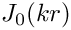, where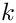is a wavenumber and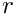is the radial spatial coordinate. This function has the property that its derivative with respect toevaluated atis zero, which must be the case if the interface is to be smooth at the symmetry boundary (the central axis of the cylinder).

A further consideration is that we want the equilibrium state of the system to be such that the interface is a flat line at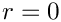, where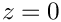is the axial coordinate. This state can only be reached if we choose an initial deformation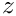which is volume conserving, and we therefore require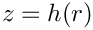to be satisfied, where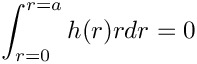is the radius of the cylindrical container (or the width of the computational domain). Since in our case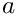, and we will choose a computational domain of width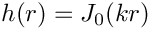, our initial condition must therefore satisfy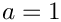Using the properties of Bessel functions we find that this condition is met if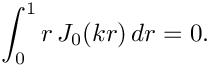, and hence the values ofare constrained to be zeroes of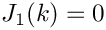.

Furthermore, the properties of Bessel functions are such that the derivative ofwith respect to, evaluated at any point along the-axis which corresponds to a zero of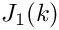, is itself zero. The velocity boundary condition in the axial direction is therefore the traction-free condition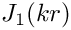. This could be physically realised by having a ‘slippery’ outer wall where the contact line can move but the contact angle is fixed at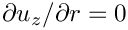, and is in fact an identical condition to the symmetry boundary condition prescribed at the axis of symmetry ().

# The example problem

We will illustrate the solution of the unsteady axisymmetric Navier–Stokes equations using the example of a distorted interface between two viscous fluids which is allowed to relax. The domain is symmetric about the line, which corresponds to the axis of the cylindrical container.

 The unsteady axisymmetric Navier–Stokes equations either side of a distorted interface. Solve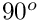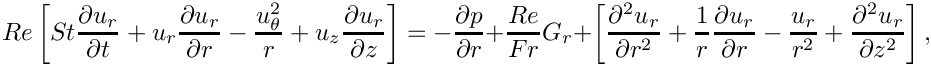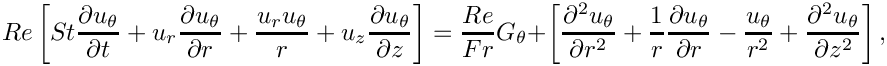and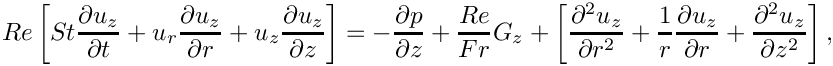in the ‘lower’ fluid, and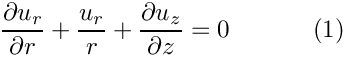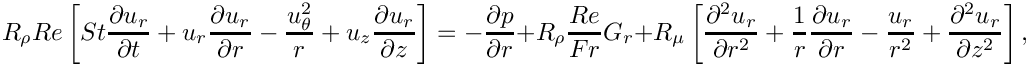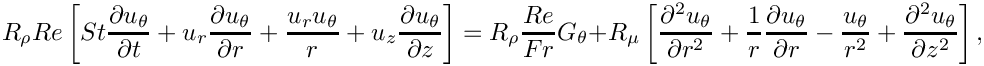and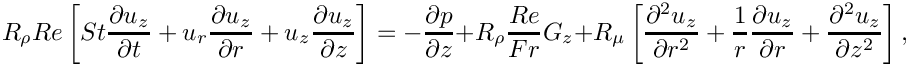in the ‘upper’ fluid. Gravity acts in the negativedirection and so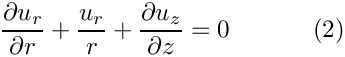and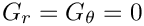. The governing equations are subject to the no slip boundary conditions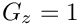on the top (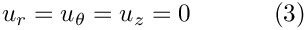) and bottom (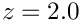) solid boundaries and the symmetry boundary conditions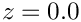on the left (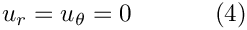) and right (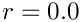) boundaries. We denote the position vector to the interface between the two fluids by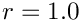, which is subject to the kinematic condition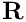and the dynamic condition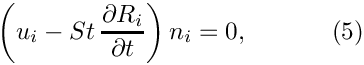The non-dimensional, symmetric stress tensor in the ‘lower’ fluid is defined as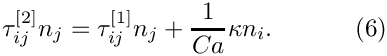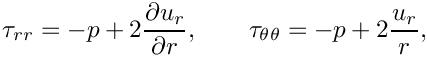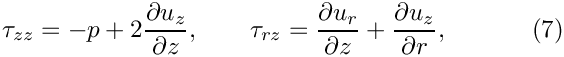and that in the ‘upper’ fluid is defined as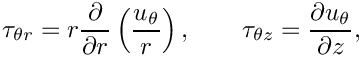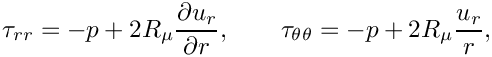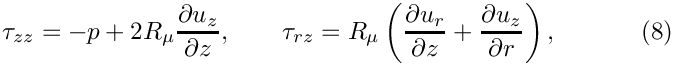The initial shape of the interface is defined by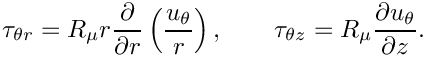where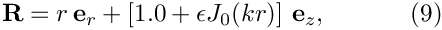is the amplitude of the initial deflection,is a wavenumber andis a zeroth-order Bessel function of the first kind.

# Results

The figure below shows a contour plot of the pressure distribution taken from an animation of the flow field, for the parameters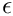,,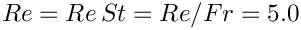and.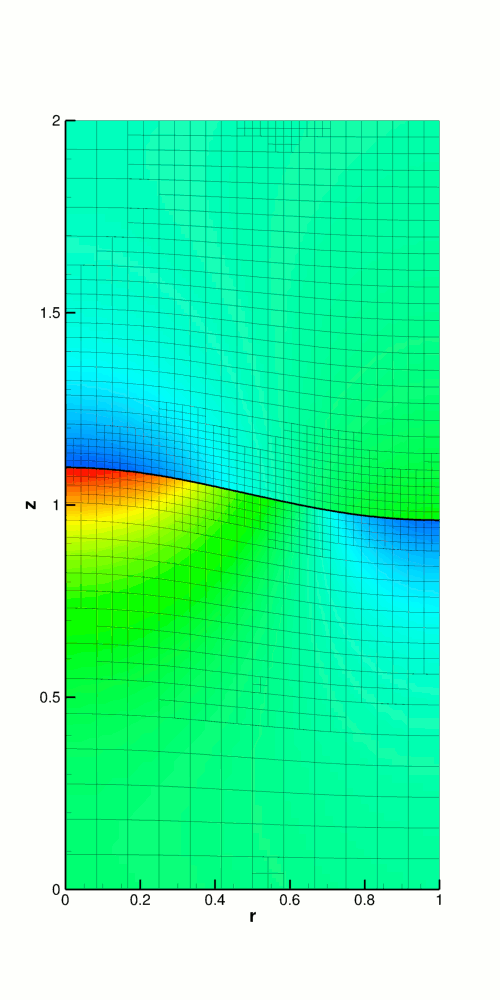Pressure contour plot for the axisymmetric relaxing interface problem.

The restoring forces of surface tension and gravitational acceleration act to revert the interface to its undeformed flat state. The interface oscillates up and down, but the motion is damped as the energy in the system is dissipated through viscous forces. Eventually the interface settles down to its equilibrium position, as can be seen in the following time-trace of the height of the interface at the left-hand edge of the domain ().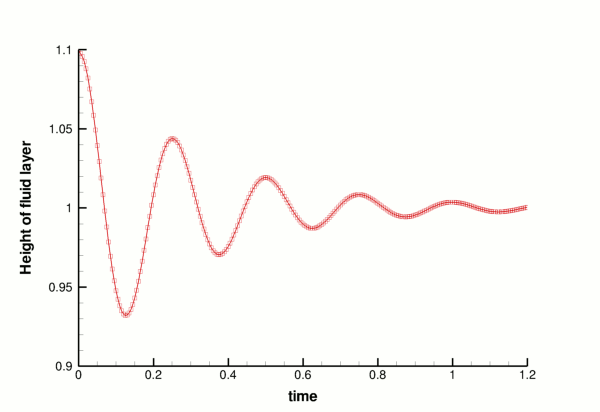Time-trace of the height of the interface at the symmetry axis (r=0).

# Validation

The free surface boundary conditions for the axisymmetric Navier–Stokes equations have been validated against an analytical test case, and we present the results in the figure below. For sufficiently small amplitudes,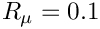, we can linearise the governing equations and obtain a dispersion relation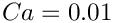. In the two-dimensional single-layer example we discussed the derivation of such a dispersion relation, and the technique used for this problem is very similar. The major difference is that the proposed separable solution for the linearised equations is of the form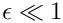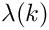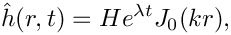and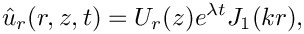As in the two-dimensional two-layer example, we will have a linear system containing nine unknowns, rather than the five unknowns that arise from a single-fluid problem. The real and imaginary parts of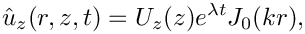correspond to the growth rate and the frequency of the oscillating interface respectively, and can be compared to numerical results computed for given values of the wavenumber. We choose an initial deflection amplitude of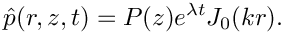and determine the growth rate and frequency of the oscillation from a time-trace of the left-hand edge of the interface.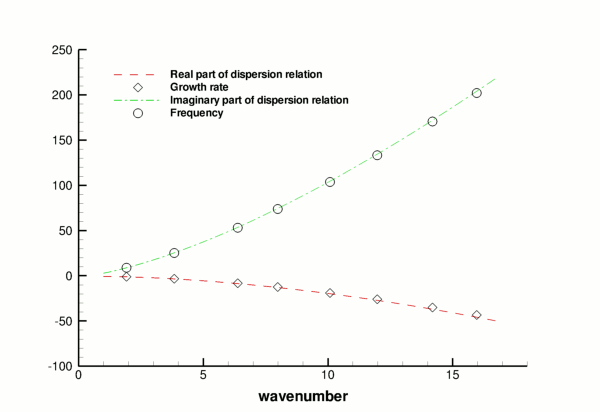Validation of the code (points) by comparison with an analytical dispersion relation (lines).

# Global parameters and functions

As in the earlier example, we use a namespace to define the dimensionless parameters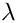,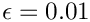,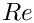and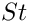. Since we are solving for the unknown bulk nodal positions by treating the interior of the mesh as a fictitious elastic solid, we also need to define the Poisson ratio for this ‘pseudo-solid's’ generalised Hookean constitutive law,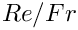. As before, we define the density and viscosity ratios of the top fluid to the bottom fluid and a unit vector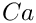which points in the direction of gravity. The only difference from before is that in this casemust have three dimensions since this is an axisymmetric problem.

/// Direction of gravity
Vector<double> G(3);
Vector< double > G(3)
Direction of gravity.

# The driver code

The first section of the driver code is identical to that of the earlier example. We define a command line flag which allows us to run a ‘validation’ version of the code (for oomph-lib's self-testing routines) and check that the non-dimensional quantities provided in the Global_Physical_Variables namespace are self-consistent. Next we specify the duration of the simulation and the size of the timestep. If we are running the code as a validation, we set the length of the simulation such that only two timesteps are taken. The direction in which gravity acts is defined to be vertically downwards.

// Set direction of gravity (vertically downwards)

Finally, we build the problem using the ‘pseudo-solid’ version of RefineableAxisymmetricQCrouzeixRaviartElements and the BDF<2> timestepper, before calling unsteady_run(...). This function solves the system at each timestep using the Problem::unsteady_newton_solve(...) function before documenting the result.

// Set up the elastic test problem with AxisymmetricQCrouzeixRaviartElements,
// using the BDF<2> timestepper
InterfaceProblem<RefineablePseudoSolidNodeUpdateElement<
RefineableAxisymmetricQCrouzeixRaviartElement,
RefineableQPVDElement<2,3> >,BDF<2> > problem;
} // End of main
/////////////////////////////////////////////////////////////////////// /////////////////////////////...
void unsteady_run(const double &t_max, const double &dt)
Do unsteady run up to maximum time t_max with given timestep dt.

# The mesh class

The mesh class is almost identical to that in the two-dimensional problem. The only difference is that, given that this (specific) mesh is to be used in an axisymmetric problem, it does not make sense to allow the mesh to be periodic in the horizontal direction.

# The problem class

The problem class is almost identical to the two-dimensional problem. The only modification arises in the arguments of deform_free_surface(...), as the initial shape of the interface is a Bessel functionas opposed to a cosine curve.

/// Deform the mesh/free surface to a prescribed function
void deform_free_surface(const double &epsilon, const double &k);

# The problem constructor

The constructor starts by building the timestepper and setting the dimensions of the mesh. The number of elements in theanddirections in both fluid layers are specified. The next section of the constructor is exactly as before. We build the bulk mesh, create an error estimator and set the maximum refinement level. An empty surface mesh is created and populated with a call to create_interface_elements, before the two meshes are combined to form the global mesh. Next we define the boundary conditions. On the top and bottom boundaries (and) we apply the no-slip condition by pinning all three velocity components. On the outer (solid) wall () we pin the radial (no penetration) and azimuthal (no slip) components but not the axial component. This simulates the "slippery" outer wall. These conditions are also applied at the symmetry boundary (). The vertical position of the nodes on the top and bottom boundaries are also pinned.

// Set the boundary conditions for this problem
// --------------------------------------------
// All nodes are free by default -- just pin the ones that have
// Dirichlet conditions here
// Determine number of mesh boundaries
const unsigned n_boundary = Bulk_mesh_pt->nboundary();
// Loop over mesh boundaries
for(unsigned b=0;b<n_boundary;b++)
{
// Determine number of nodes on boundary b
const unsigned n_node = Bulk_mesh_pt->nboundary_node(b);
// Loop over nodes on boundary b
for(unsigned n=0;n<n_node;n++)
{
// Fluid boundary conditions:
// --------------------------
// Pin radial and azimuthal velocities (no slip/penetration)
// on all boundaries other than the interface (b=4)
if(b!=4)
{
Bulk_mesh_pt->boundary_node_pt(b,n)->pin(0);
Bulk_mesh_pt->boundary_node_pt(b,n)->pin(2);
}
// Pin axial velocity on top (b=2) and bottom (b=0) boundaries
// (no penetration). Because we have a slippery outer wall we do
// NOT pin the axial velocity on this boundary (b=1); similarly,
// we do not pin the axial velocity on the symmetry boundary (b=3).
if(b==0 || b==2) { Bulk_mesh_pt->boundary_node_pt(b,n)->pin(1); }
// Solid boundary conditions:
// --------------------------
// Pin vertical displacement on solid boundaries
if(b==0 || b==2) { Bulk_mesh_pt->boundary_node_pt(b,n)->pin_position(1); }
} // End of loop over nodes on boundary b
} // End of loop over mesh boundaries

We pin the horizontal position of all nodes in the mesh as well as the azimuthal velocity components throughout the bulk of the domain (since in an axisymmetric problem these should remain zero always).

// Loop over all nodes in mesh
const unsigned n_node = Bulk_mesh_pt->nnode();
for(unsigned n=0;n<n_node;n++)
{
// Pin horizontal displacement of all nodes
Bulk_mesh_pt->node_pt(n)->pin_position(0);
// Pin all azimuthal velocities throughout the bulk of the domain
Bulk_mesh_pt->node_pt(n)->pin(2);
}

The remainder of the problem constructor is identical to that of the two-dimensional problem. We create a generalised Hookean constitutive equation for the pseudo-solid mesh before looping over all elements in the bulk mesh and assigning pointers to the Reynolds and Womersley numbers,and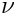, the product of the Reynolds number and the inverse of the Froude number,, the direction of gravity,, the constitutive law and the global time object. For those elements which correspond to the upper fluid layer, we also assign pointers for the viscosity and density ratios,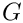and. We pin one degree of freedom and call the function which assigns the boundary conditions, before finally setting up the equation numbering scheme.

# Initial conditions

The set_initial_conditions() function is very similar to that in the two-dimensional problem. We loop over all nodes in the mesh and set all three velocity components to zero, before calling Problem::assign_initial_values_impulsive(). This function copies the current nodal values and positions into the required number of history values for the timestepper in question, simulating an impulsive start.

//==start_of_set_initial_condition========================================
/// Set initial conditions: Set all nodal velocities to zero and
/// initialise the previous velocities and nodal positions to correspond
/// to an impulsive start
//========================================================================
template <class ELEMENT, class TIMESTEPPER>
{
// Determine number of nodes in mesh
const unsigned n_node = Bulk_mesh_pt->nnode();
// Loop over all nodes in mesh
for(unsigned n=0;n<n_node;n++)
{
// Loop over the three velocity components
for(unsigned i=0;i<3;i++)
{
// Set velocity component i of node n to zero
Bulk_mesh_pt->node_pt(n)->set_value(i,0.0);
}
}
// Initialise the previous velocity values and nodal positions
// for timestepping corresponding to an impulsive start
assign_initial_values_impulsive();
} // End of set_initial_condition
void set_initial_condition()
Set initial conditions.

# Boundary conditions

The set_boundary_conditions() function is very similar to that in the two-dimensional problem.

//==start_of_set_boundary_conditions======================================
/// Set boundary conditions: Set all velocity components to zero
/// on the top and bottom (solid) walls and the radial and azimuthal
/// components only to zero on the side boundaries
//========================================================================
template <class ELEMENT, class TIMESTEPPER>
{
// Determine number of mesh boundaries
const unsigned n_boundary = Bulk_mesh_pt->nboundary();
// Loop over mesh boundaries
for(unsigned b=0;b<n_boundary;b++)
{
// Determine number of nodes on boundary b
const unsigned n_node = Bulk_mesh_pt->nboundary_node(b);
// Loop over nodes on boundary b
for(unsigned n=0;n<n_node;n++)
{
// Set radial component of the velocity to zero on all boundaries
// other than the interface (b=4)
if(b!=4) { Bulk_mesh_pt->boundary_node_pt(b,n)->set_value(0,0.0); }
// Set azimuthal component of the velocity to zero on all boundaries
// other than the interface (b=4)
if(b!=4) { Bulk_mesh_pt->boundary_node_pt(b,n)->set_value(2,0.0); }
// Set axial component of the velocity to zero on solid boundaries
if(b==0 || b==2)
{
Bulk_mesh_pt->boundary_node_pt(b,n)->set_value(1,0.0);
}
}
}
} // End of set_boundary_conditions
void set_boundary_conditions()
Set boundary conditions.

# Actions before and after adaptation

These functions follow exactly the same structure as those in the two-dimensional problem.

# Create interface elements

This function is identical to that in the two-dimensional problem, with the exception that instead of creating interface elements of the type

ElasticLineFluidInterfaceElement<ELEMENT>,

we are creating interface elements of the type

ElasticAxisymFluidInterfaceElement<ELEMENT>,

where ELEMENT is the templated bulk element type.

# Delete interface elements

This function is identical to that in the two-dimensional problem.

# Prescribing the initial free surface position

At the beginning of the simulation the interface is deformed by a prescribed function (9), implemented in the function deform_free_surface(...), which cycles through the bulk mesh's Nodes and modifies their positions such that the nodes on the free surface follow the prescribed interface shape and the bulk nodes retain their fractional position between the solid boundaries and the (now deformed) interface.

//==start_of_deform_free_surface==========================================
/// Deform the mesh/free surface to a prescribed function
//========================================================================
template <class ELEMENT, class TIMESTEPPER>
deform_free_surface(const double &epsilon,const double &k)
{
// Initialise Bessel functions (only need the first!)
double j0, j1, y0, y1, j0p, j1p, y0p, y1p;
// Determine number of nodes in the "bulk" mesh
const unsigned n_node = Bulk_mesh_pt->nnode();
// Loop over all nodes in mesh
for(unsigned n=0;n<n_node;n++)
{
// Determine eulerian position of node
const double current_r_pos = Bulk_mesh_pt->node_pt(n)->x(0);
const double current_z_pos = Bulk_mesh_pt->node_pt(n)->x(1);
// Compute Bessel functions
CRBond_Bessel::bessjy01a(k*current_r_pos,j0,j1,y0,y1,j0p,j1p,y0p,y1p);
// Determine new vertical position of node
const double new_z_pos = current_z_pos
+ (1.0-fabs(1.0-current_z_pos))*epsilon*j0;
// Set new position
Bulk_mesh_pt->node_pt(n)->x(1) = new_z_pos;
}
} // End of deform_free_surface
void deform_free_surface(const double &epsilon, const double &k)
Deform the mesh/free surface to a prescribed function.

# Post-processing

This function follows an identical structure to that in the two-dimensional problem.

# The timestepping loop

The function unsteady_run(...) is used to perform the timestepping procedure, and is very similar to that in the two-dimensional problem. The only difference arises from the fact that the initial interface deformation is a Bessel function rather than a cosine curve.

// Set value of epsilon
const double epsilon = 0.1;
// Set value of k in Bessel function J_0(kr)
const double k_bessel = 3.8317;
// Deform the mesh/free surface
deform_free_surface(epsilon,k_bessel);

The rest of the function is identical to before.